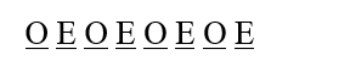# How many words can be formed out of the letters of the word ‘ORIENTAL’

Question:

How many words can be formed out of the letters of the word ‘ORIENTAL’ so that the vowels always occupy the odd places?

Solution:

To find: number of words formed

Condition: vowels occupy odd places

There are 8 letters in the word ORIENTAL and vowels are 4 which are O, I, E,A respectively.There is 4 odd places in which 4 vowels are to be arranged.

The rest 4 letters can be arranged in $4 !$ Ways.

Formula:

Number of permutations of $n$ distinct objects among $r$ different places, where repetition is not allowed, is

$P(n, r)=n ! /(n-r) !$

Therefore, the total arrangement is

$P(4,4) \times 4 !=\frac{4 !}{(4-4) !} \times 4 !=\frac{4 !}{0 !} \times 4 !=\frac{24}{1} \times 24=576$

Therefore, total number of words formed are 576